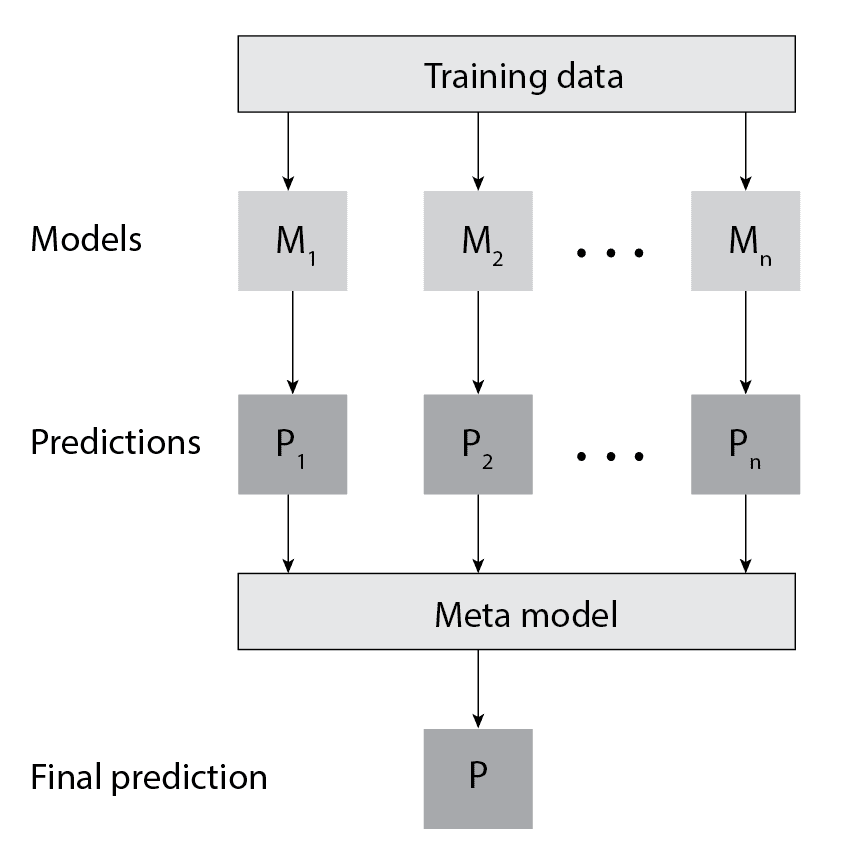## 1. Intro

In the machine learning field, there are many technical terms that contain the word “learning”. Some of them are deep learning, reinforcement learning, supervised or unsupervised learning, active learning, meta-learning, and transfer learning. Despite the common word “learning”, these terms are very different. The only common thing between them is that we use them in machine learning.

For people who are not actively involved in machine learning or for someone who just started to research this field, all these terms might sound very similar and create confusion. To clarify them a bit, in this tutorial, we’ll explain the terms meta-learning and transfer learning following with some examples.

## 2. Transfer Learning

Transfer learning is a very popular technique in deep learning where existing pre-trained models are used for new tasks. Basically, the idea is to take one neural network that we trained for one specific task and use it as a starting point for another task. Sometimes, when we need to use an existing neural network for a different task, for example, the classification of three classes instead of two, it is possible to change only a few last layers of the network and to keep the rest as it is.

Nowadays, in computer vision and natural language processing fields, researchers and engineers use huge neural networks that require vast computing and time resources to learn. Instead of retraining these models every time, thanks to transfer learning, we can take these models as starting points for our tasks and fine-tune them a bit for our purpose. Of course, the more similar the original training data is to our data set, the less fine-tuning we need to do.

### 2.1. Why Does Transfer Learning Work?

Transfer learning is possible because neural networks tend to learn different patterns in the data. For example, in computer vision, initial layers learn low-level features such as lines, dots, and curves. Top layers learn high-level features built on top of low-level features. Most of the patterns, especially low-level ones, are common to many different computer image data sets. Instead of learning them every time from scratch, we can use existing parts of the network and reuse them with a bit of tweaking for our purposes.

## 3. Meta-Learning

The word “meta” usually indicates something more comprehensive or more abstract. For example, a metaverse is a virtual world or the world inside our world, metadata is data that provides information about other data and similarly.

Likewise, in this case, meta-learning refers to learning about learning. Meta-learning includes machine learning algorithms that learn from the output of other machine learning algorithms.

Commonly, in machine learning, we try to find what algorithms work best with our data. These algorithms learn from historical data to produce models and those models can be used later to predict outputs for our tasks. Meta-learning algorithms don’t use directly that kind of historic data but they learn from the outputs of machine-learning models. This means that meta-learning algorithms require the presence of other models that have already been trained on data.

For example, if the goal is to classify images, machine learning models take images as input and predict classes while meta-learning models take predictions of those machine learning models as input and based on that, predict classes of the images. In that sense, meta-learning occurs one level above machine learning.

In order to explain the concept of meta-learning more intuitively, we will mention a few examples below.

### 3.1. Examples of Meta-Learning

Probably, the most popular meta-learning technique is stacking. Stacking is a type of ensemble learning algorithm that combines the results of two or more machine learning models. It learns how to best combine multiple predictions into one.

The idea is to train individual models that are part of an ensemble model using training data. Then, predictions of those models are used as input to the meta-model. In this case, those predictions became meta-features for the meta-model. For stacking models, it is important to use cross-validation techniques to prevent overfitting:Besides that, there are many other types of meta-learning algorithms. Some of them are:

• Meta-learning optimizers – they optimize existing neural networks to learn faster with new data.
• Metric meta-learning – with the goal of learning an embedding for neural networks where the distance between similar input samples becomes closer and vice versa for dissimilar samples.

## 4. Conclusion

In this article, we gave a brief explanation of the concepts of transfer learning and meta-learning. In one sentence, transfer learning is a technique of reusing existing neural networks and on the other hand, meta-learning is an idea of learning about learning.